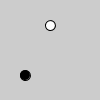Name

map()

Examples# Map between positive values.
value = 50
m = map(value, 0, width, 0, width/2)
# Draw white ellipse at original x location.
ellipse(value, height *.25, 10, 10)
# Draw black ellipse at mapped x location
fill(0)
ellipse(m, height*.75, 10, 10)
value = 110
m = map(value, 0, 100, -20, -10)
print(m)# Prints "-9.0"
def setup():
size(200, 200)
noStroke()

def draw():
background(204)
x1 = map(mouseX, 0, width, 50, 150)
ellipse(x1, 75, 50, 50)
x2 = map(mouseX, 0, width, 0, 200)
ellipse(x2, 125, 50, 50)
Description Re-maps a number from one range to another.

In the first example above, the number 50 is converted from a value in the range of 0 to the width of the window (which is the default value of 100) into a value that ranges from the left edge of the window (0) to half the width of the window (50).

As shown in the second example, numbers outside of the range are not clamped to the minimum and maximum parameters values, because out-of-range values are often intentional and useful.

The third example shows how this function may be applied to user input.
Syntax
map(value, start1, stop1, start2, stop2)
Parameters
value float: the incoming value to be converted float: lower bound of the value's current range float: upper bound of the value's current range float: lower bound of the value's target range float: upper bound of the value's target range
Related norm()
lerp()

Updated on Mon Sep 21 15:53:25 2020.

If you see any errors or have comments, please let us know.